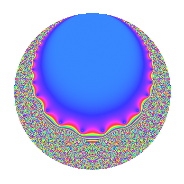# Properties

 Label 16.12.a.dLevel 16 Weight 12 Character orbit 16.a Self dual Yes Analytic conductor 12.293 Analytic rank 0 Dimension 2 CM No Inner twists 1

# Related objects

## Newspace parameters

 Level: $$N$$ = $$16 = 2^{4}$$ Weight: $$k$$ = $$12$$ Character orbit: $$[\chi]$$ = 16.a (trivial)

## Newform invariants

 Self dual: Yes Analytic conductor: $$12.293490889$$ Analytic rank: $$0$$ Dimension: $$2$$ Coefficient field: $$\Q(\sqrt{109})$$ Coefficient ring: $$\Z[a_1, a_2, a_3]$$ Coefficient ring index: $$2^{7}$$ Fricke sign: $$1$$ Sato-Tate group: $\mathrm{SU}(2)$

## $q$-expansion

Coefficients of the $$q$$-expansion are expressed in terms of $$\beta = 64\sqrt{109}$$. We also show the integral $$q$$-expansion of the trace form.

 $$f(q)$$ $$=$$ $$q + ( -28 - \beta ) q^{3} + ( 3934 - 12 \beta ) q^{5} + ( -45528 - 42 \beta ) q^{7} + ( 270101 + 56 \beta ) q^{9} +O(q^{10})$$ $$q + ( -28 - \beta ) q^{3} + ( 3934 - 12 \beta ) q^{5} + ( -45528 - 42 \beta ) q^{7} + ( 270101 + 56 \beta ) q^{9} + ( -79540 + 693 \beta ) q^{11} + ( 525238 + 948 \beta ) q^{13} + ( 5247416 - 3598 \beta ) q^{15} + ( 715442 - 10824 \beta ) q^{17} + ( 10933300 + 4587 \beta ) q^{19} + ( 20026272 + 46704 \beta ) q^{21} + ( -17903368 + 24738 \beta ) q^{23} + ( 30939047 - 94416 \beta ) q^{25} + ( -27604696 - 94522 \beta ) q^{27} + ( -114413850 + 59556 \beta ) q^{29} + ( -32361056 + 28632 \beta ) q^{31} + ( -307172432 + 60136 \beta ) q^{33} + ( 45910704 + 381108 \beta ) q^{35} + ( 37779390 - 81564 \beta ) q^{37} + ( -437954536 - 551782 \beta ) q^{39} + ( 600607098 + 647088 \beta ) q^{41} + ( 22759916 - 324051 \beta ) q^{43} + ( 762553526 - 3020908 \beta ) q^{45} + ( 614539632 + 816420 \beta ) q^{47} + ( 883034537 + 3824352 \beta ) q^{49} + ( 4812493960 - 412370 \beta ) q^{51} + ( -1904274962 + 3514980 \beta ) q^{53} + ( -4025704984 + 3680742 \beta ) q^{55} + ( -2354062768 - 11061736 \beta ) q^{57} + ( 3006463292 - 4307463 \beta ) q^{59} + ( 4894896454 + 4588692 \beta ) q^{61} + ( -13347241656 - 13893810 \beta ) q^{63} + ( -3012688172 - 2573424 \beta ) q^{65} + ( -7351547612 + 21317583 \beta ) q^{67} + ( -10543332128 + 17210704 \beta ) q^{69} + ( -2159995544 + 20099142 \beta ) q^{71} + ( 5527819738 - 3259368 \beta ) q^{73} + ( 41287051708 - 28295399 \beta ) q^{75} + ( -9373484064 - 28210224 \beta ) q^{77} + ( -25978811632 - 41373444 \beta ) q^{79} + ( -4873980151 + 20331080 \beta ) q^{81} + ( 54113987956 + 13552251 \beta ) q^{83} + ( 60804864860 - 51166920 \beta ) q^{85} + ( -23386022184 + 112746282 \beta ) q^{87} + ( 35594145930 + 90758808 \beta ) q^{89} + ( -41689446288 - 65220540 \beta ) q^{91} + ( -11877047680 + 31559360 \beta ) q^{93} + ( 18436437784 - 113154342 \beta ) q^{95} + ( -849903838 - 64199400 \beta ) q^{97} + ( -4157458628 + 182725753 \beta ) q^{99} +O(q^{100})$$ $$\operatorname{Tr}(f)(q)$$ $$=$$ $$2q - 56q^{3} + 7868q^{5} - 91056q^{7} + 540202q^{9} + O(q^{10})$$ $$2q - 56q^{3} + 7868q^{5} - 91056q^{7} + 540202q^{9} - 159080q^{11} + 1050476q^{13} + 10494832q^{15} + 1430884q^{17} + 21866600q^{19} + 40052544q^{21} - 35806736q^{23} + 61878094q^{25} - 55209392q^{27} - 228827700q^{29} - 64722112q^{31} - 614344864q^{33} + 91821408q^{35} + 75558780q^{37} - 875909072q^{39} + 1201214196q^{41} + 45519832q^{43} + 1525107052q^{45} + 1229079264q^{47} + 1766069074q^{49} + 9624987920q^{51} - 3808549924q^{53} - 8051409968q^{55} - 4708125536q^{57} + 6012926584q^{59} + 9789792908q^{61} - 26694483312q^{63} - 6025376344q^{65} - 14703095224q^{67} - 21086664256q^{69} - 4319991088q^{71} + 11055639476q^{73} + 82574103416q^{75} - 18746968128q^{77} - 51957623264q^{79} - 9747960302q^{81} + 108227975912q^{83} + 121609729720q^{85} - 46772044368q^{87} + 71188291860q^{89} - 83378892576q^{91} - 23754095360q^{93} + 36872875568q^{95} - 1699807676q^{97} - 8314917256q^{99} + O(q^{100})$$

## Embeddings

For each embedding $$\iota_m$$ of the coefficient field, the values $$\iota_m(a_n)$$ are shown below.

For more information on an embedded modular form you can click on its label.

Label $$\iota_m(\nu)$$ $$a_{2}$$ $$a_{3}$$ $$a_{4}$$ $$a_{5}$$ $$a_{6}$$ $$a_{7}$$ $$a_{8}$$ $$a_{9}$$ $$a_{10}$$
1.1
 5.72015 −4.72015
0 −696.180 0 −4084.16 0 −73591.5 0 307519. 0
1.2 0 640.180 0 11952.2 0 −17464.5 0 232683. 0
 $$n$$: e.g. 2-40 or 990-1000 Significant digits: Format: Complex embeddings Normalized embeddings Satake parameters Satake angles

## Inner twists

This newform does not admit any (nontrivial) inner twists.

## Atkin-Lehner signs

$$p$$ Sign
$$2$$ $$1$$

## Hecke kernels

This newform can be constructed as the kernel of the linear operator $$T_{3}^{2} + 56 T_{3} - 445680$$ acting on $$S_{12}^{\mathrm{new}}(\Gamma_0(16))$$.